# 2. Semiconductor Physics

## 2.1 Basic Band Theory

### 2.1.1 Essentials of the Free Electron GasThis course requires that you must be familiar with some solid state physics including a working knowledge of thermodynamics and quantum theory.The free electron gas model is a paradigm for the behavior of electrons in a crystal, you should be thoroughly familiar with it.In case of doubt, refer to the Hyperscript "MaWi II" – which, however, is in German.In the following, the essentials of the model are repeated – briefly, without much text. If you have serious problems with the topic already here, you do indeed have a problem with this course!

The Energy Levels of an Electron in a Constant PotentialThe free electron gas model works with a constant potential. This is, of course, a doubtful approximation; essentially only justified because it works – up to a point.Approximations: Constant potential U = U0 = 0 within a crystal with length L in all directions; U = ¥ outside; only one electron is considered.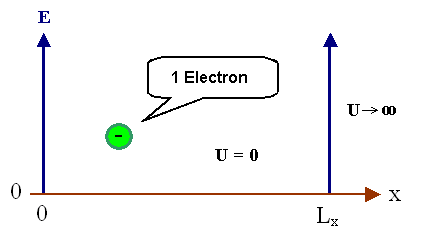Graphic representation of the model. Note that the electron energy inside the potential well can only be purely kinetic.The major formulas and interpretations needed are:Time independent one-dimensional Schrödinger equation :
–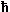2 2me æ ç è ö ÷ ø= h "bar" = h/2p = Plancks constant/2p
me = electron mass
y = wave function
E = total energy = kinetic energy + potential energy. Here it is identical to the kinetic energy because the potential energy is zero.Potential U(x) as defined above; i.e. U(x) = U0 = const = 0 for 0  £  x  £  L, or ¥ otherwise. (Remember that L is the macroscopic size of the crystal.)For the (potential) energy, there is always a free choice of zero point; here it is convenient to put the bottom of the potential well at zero potential energy. We will, however, change that later on.Boundary conditions: Several choices are possible! Here, we use periodic conditions (also called Bornvon Karman conditions), leading to a so-called supercell:
y(x + L)  =  y(x)Solution (for 3-dimensional case)

y  = æ ç è ö ÷ ø

r  =  position vector
=  (x, y, z)

k  =  wavevector
=  (kx, ky, kz)

kx  =  ± nx · 2p/L
ky  =  ± ny · 2p/L
kz  =  ± nz · 2p/L

nx, ny, nz  =  quantum numbers
=  0, 1, 2, 3, ...

i  =  (–1)1/2A somewhat more general form for crystals with unequal sides can be found in the link.There are infinitely many solutions, and every individual solution is selected or described by a set of the three quantum numbers nx, ny, nz. The solution y describes a plane wave with amplitude (1/L)3/2 moving in the direction of the wave vector k.Next, we extract related quantities of interest in connection with moving particles or waves:The wavelength l of the "electron wave" is given by
l  =  2p
|k|
=  2p
kThe momentum p of the electron is given by
p  =· kFrom this and with m = electron mass we obtain the velocity v of the electron to be
v  =  p
me
=· k
meThe numbers nx, ny, nz are quantum numbers; their values (together with the value of the spin) are characteristic for one particular solution of the Schrödinger equation of the system. A unique set of quantum numbers (alway plus one of the two possibilities for the spin) describes a state of the electronSince these quantum numbers only appear in the wave vector k, one often denotes a particular wave function by indexing it with k instead of nx, y, z because a given k vector denotes a particular solution or state just as well as the set of the three quantum numbers.
ynx, ny, nz(x,y,z)  =  yk(x,y,z)  =  yk(r)In other words, in a formal, more abstract sense we can regard the wave vector as a kind of vector quantum number designating a special solution of the Schrödinger equation for the given problem.Since in the present case the total energy E is identical to the kinetic energy Ekin = ½mev2 = ½p2/me, we have
E  =2 · k2
2meWe now have expressed the total energy as a function of the wave vector. Any relation of this kind is called a dispersion function. Spelt out we have
E  =2   · 2me æ ç è ö ÷ ø æ è ö øThis is the first important result: There are only discrete energy levels for the electron in a box with constant potential that represents the crystal.This result (as you simply must believe at this point) will still be true if we use the correct potentials, and if we consider many electrons. The formula, however, i.e. the relation between energy and wave vectors may become much more complicated.The boundary conditions chosen and the length L of the box are somewhat arbitrary. We will see, however, that they do not matter for the relevant quantities to be derived from this model.

Density of StatesKnowing the energy levels, we can count how many energy levels are contained in an interval DE at the energy E. This is best done in k-space or phase space.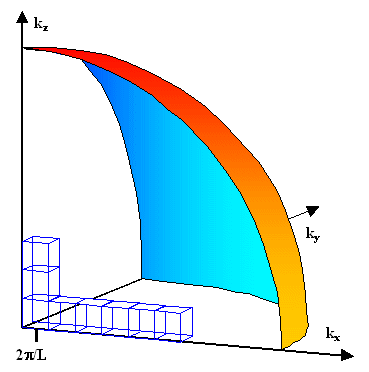For the free electron gas, in phase space a surface of constant energy is a sphere, as schematically shown in the picture.Any "state", i.e. solution of the Schroedinger equation with a specific k, occupies the volume given by one of the little cubes in phase space, corresponding to the discrete states just discussed.The number of little cubes fitting inside the sphere at energy E thus is the number of all electronic states y up to E.Since every state (characterized by its set of quantum numbers nx, ny, nz) can accommodate 2 electrons (one with spin up, one with spin down), the total number of electrons that can occupy states up to E is twice the number of little cubes; let this number (that includes the spin degeneracy) be Ns(E).Looking just at the energy, it is clear that at higher energies there are more states available. Counting the number of little cubes just in an energy interval E, E + DE corresponds to taking the difference of the numbers of cubes contained in a sphere with "radius" E + DE and E.We thus obtain the density of states D(E) as
D(E)  =  1
V
·  Ns(E + DE) – Ns(E)
DE
=   1
V
·  dNs
dE
=     1
L3
·  dNs
dENote that D(E) is a density both with respect to E and to V = L3 (= volume of the crystal).The final formula is
D(E)  =   1 L3 æ ç è ö ÷ øThe derivation of this formula and more to densities of states (including generating some numbers) can be found in the link.Some important points are:• D(E) is proportional to E1/2.
• For different (but physically meaningful) boundary conditions we obtain the same D (see the exercise 2.1 below).
• The artificial length L disappears because we are only considering specific quantities, i.e. volume densities.
• D is kind of a twofold density: It is first the density of energy states in an energy interval and second the (trivial) density of that number in space.If we fill the available states with the available electrons at a temperature of 0 K (since we consider the free electrons of a material this number will be about 1 [or a few] per atom and thus is principally known) starting from E = 0, we find a special energy called Fermi energy EF at the value where the last electron finds its place.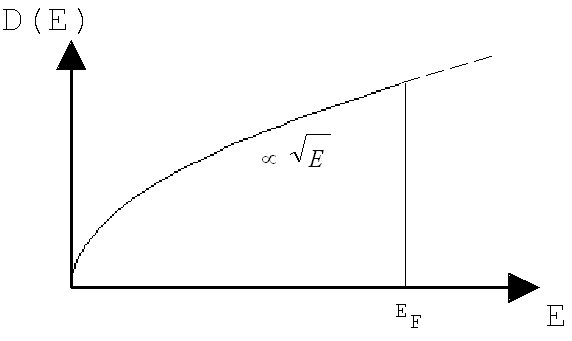In order to get (re)acquainted with the formalism, we do two simple exercises:
 Exercise 2.1-1 Solution of the free electron gas problem with fixed boundary conditions
 Exercise 2.1-2 Density of states as a function of dimensionality

Carrier StatisticsWe have the number of energy states for a given energy interval and want to know how many (charge) carriers we will find in the same energy interval in thermal equilibrium. Since we want to look at particles other than electrons too, but only at charged particles, we use the term "carrier" here.In other words, we want the distribution of carriers on the available energy levels satisfying three conditions:The Pauli exclusion principle: There may be at most 2 carries per energy state (one with spin "up", one with spin "down"), not more.The equilibrium condition: Minimum of the appropriate thermodynamic potential, here always the free Enthalpy G (also called Gibbs energy).The conservation of particles (or charge) condition; i.e.constant number of carriers regardless of the distribution.The mathematical procedure involves a variation principle of G. The result is the well-known FermiDirac distribution f(E,T):f(E,T) = probability for occupation of (one!) state at E for the temperature T
f(E, T)  =

1
exp æ
è
EEF
kT
ö
ø
+  1If you are not very familiar with the distributions in general or the Fermi distribution in particular, read up on it in the (German) link.This is the "popular" version with the Fermi energy EF as a parameter. In the "correct" version, we would have the chemical potential m instead of EF.Since the Fermi energy is a quantity defined independently of the equilibrium considerations above, equating EF with m is only correct at T = 0 K. Most textbooks emphasize that small differences may occur at larger temperatures, but do not explain what those differences are. We, like everybody else, will ignore these fine points and use the term "Fermi Energy" without reservations.In all experience, many students (and faculty) of physics or materials science have problems with the concept of the "chemical potential". This is in part psychological (we want to do semiconductor physics and not chemistry), but mostly just due to little acquaintance with the subject. The link provides some explanations and examples which might help.The Fermi-Dirac distribution has some general properties which are best explained in a graphic representation.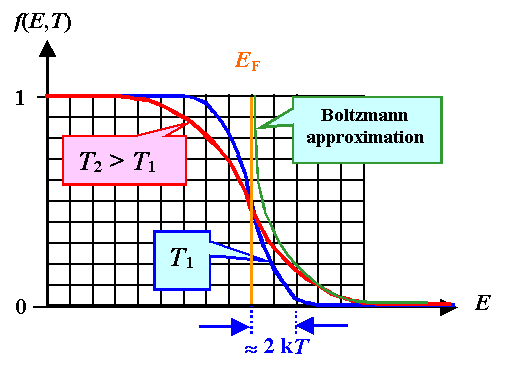It contains a convenient definition of the Fermi energy: The energy where exactly half of the available levels are occupied (or would be occupied if there would be any!) is the Fermi energy:
f(E = EF)   =  1
2The width of "soft zone" is » 4 kT = 1 meV at 3 K, and 103 meV at 300K.For EEF >> kT the Boltzmann approximation can be used:
fB(E,T)  »  exp – æ è ö øIn this case the exclusion principle is not important because there are always plenty of free states around – the electrons behave akin to classical particles.This leads to the final formula for the incremental number or density of electrons, dn, in the energy interval E, E + DE (and, of course, in thermodynamic equilibrium).
In words: Formula
Density of electrons in the energy interval E, E + DE =
density of states times probability for occupancy
times energy interval
dn  =  D(E) · f(E,T) · dEThis is an extremely important formula, which is easily generalized for almost everything. The number (or density) of something is given by the density of available places times the probability of occupation.This applies to the number of people found in a given church or stadium, the number of photons inside a "black box", the number of phonons in a crystal, and so on.The tricky part, of course, is to know the probabilities or the distribution function in each case. However, if we do not consider church goers or soccer fans, but only physical particles (including electrons and holes, but also "quasi-particles" like phonons, excitons, ...), there are only two distribution functions (and the Boltzmann distribution as an approximation): The Fermi-Dirac distribution for Fermions, and the Bose-Einstein distribution for Bosons. Mother nature here made life real easy for physicists.Since all available electrons must be somewhere on the energy scale, we always have a normalization condition for the total electron density n:
n ¥ ó õ 0

 Questions Quick Questions to 2.1.1

© H. Föll (Semiconductors - Script)